# Mathdoku Rules

### A brief guide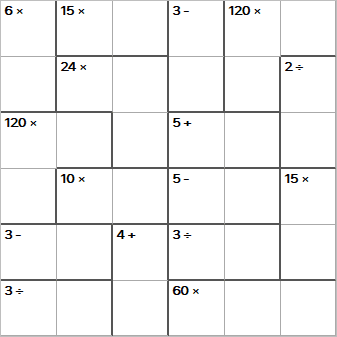The rules of Mathdoku are based on the rules of Sudoku. This is an example Mathdoku starting grid.
A completed Mathdoku grid is like a Sudoku in that each row and column must contain the numbers 1-6 once and only once (notice that this grid is smaller than the standard Sudoku grid).
In addition, the grid is split up in to multiple sections, typically between two and four cells. Each section has a number and a Mathematical symbol. You must make that number by combining the numbers with the mathematical symbol.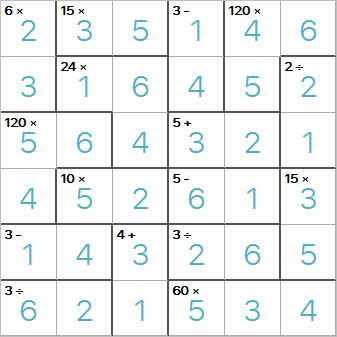This is the same grid as above, but completed. You can see that by combining the numbers with the mathematical symbol, you arrive at the target number.
As an example, the top-left block has two cells with the clue '6x', so this means you need to make 6 by multiplying the two numbers, and 2x3 does make 6!
With + and x, it doesn't matter which way round your numbers go. However, with ÷ and -, the order does matter. For Mathdoku puzzles, you can choose which way round the numbers go. So, in the bottom left we have the clues '3 -' and '3 ÷', it doesn't matter which way round the two numbers go in the cells. (It does of course matter for the general 'Sudoku' rule that each row/column must contain each number once and only once).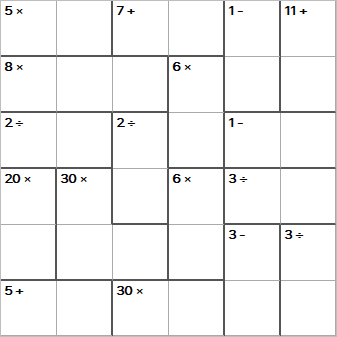This is another example starting grid. We can't fill any numbers in immediately, and this is always the case with Mathdoku puzzles.
The first step with a Mathdoku puzzle is always to enter pencil marks for those cells that don't have many combinations. For example, the top-left cell with the clue '5x' is a great example. There is only way to satisfy that clue, i.e. 1x5 = 5. So we can enter 1 and 5 for the pencil marks for those two cells.
There are two ways to satisfy '6x', namely 1x6 = 6, and 2x3 = 6, so it's not worth looking at that block.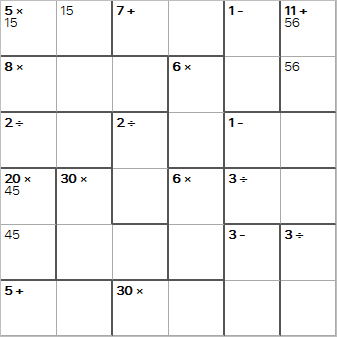We have now entered some of the pencil marks, and we will use the 'naked pairs' technique here. For the column on the far-left, we have a pair of cells with the clue '20x', and we have the possibilities of 4 and 5. The 4 and 5 in this column must go in those two cells, we don't know which way round yet, but they must go in those two cells.
This means the top-left cell can't be a 5, and therefore must be a 1.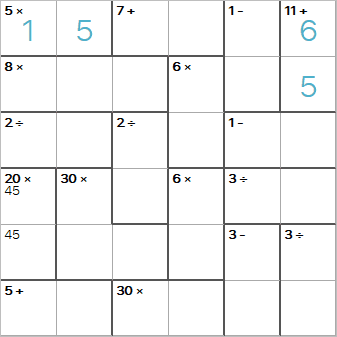There are a further 3 cells we can now fill in, and this is quite often the case with Mathdoku puzzles.
Having filled this in, we can now look at the two cells in the top row with '7+' as the clue. We can't use 1, 5 or 6 again in this top row, so we can only make '7+' with 3+4, which means the last cell in this row must be a 2.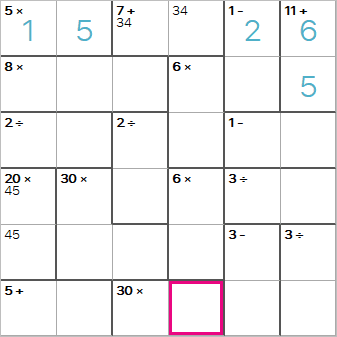We have now made good progress in solving this Mathdoku, and you have just learnt the rules you need to be able to solve a Mathdoku puzzle.
You should now be able to work out the value that goes in the highlighted cell.